Before understanding what is an adjoint of a matrix, let’s recall what is a matrix and related terms. In linear algebra, a matrix is an ordered rectangular array of numbers or functions. The numbers or functions are called the elements or the entries of the matrix. A matrix having m rows and n columns is called a matrix of order m × n or m × n matrix. However, matrices can be classified based on the number of rows and columns in which elements are arranged. In this article, you will learn about the adjoint of a matrix, finding the adjoint of different matrices, and formulas and examples.

## Adjoint of a Matrix Definition

The adjoint of a square matrix $A=[a_{ij}]_{n\times n}$ is defined as the transpose of the matrix $[A_{ij}]_{n\times n}$, where $A_{ij}$ is the cofactor of the element $a_{ij}$. In other words, the transpose of a cofactor matrix of the square matrix is called the adjoint of the matrix. Adjoint of the matrix A is denoted by $adj\ A$. This is also known as adjugate matrix or adjunct matrix.

It is necessary to find the adjoint of a given matrix to calculate the inverse matrix. This can be done only for square matrices.

## Adjoint of a Matrix Formula

Formula for the adjoint of a matrix can be derived using the cofactor and transpose of a matrix. However, it is easy to find the adjugate matrix for a 2 x 2 matrix. Let’s have a look at the formulas and procedure of finding the adjoint matrix for a given matrix.

## Adjoint Of a Matrix 2 x 2

Let A be the 2 x 2 matrix and is given by:

$\large A=\begin{bmatrix} a_{11} & a_{12}\\ a_{21} & a_{22} \end{bmatrix}$

Then, the adjoint of this matrix is:

$\large adj\ A=\begin{bmatrix} A_{11} & A_{21}\\ A_{12} & A_{22} \end{bmatrix}$

Here,

A11 = Cofactor of a11

A12 = Cofactor of a12

A21 = Cofactor of a21

A22 = Cofactor of a22

Alternatively, the adj A can also be calculated by interchanging a11 and a22 and by changing signs of a12 and a21. This can be shown as: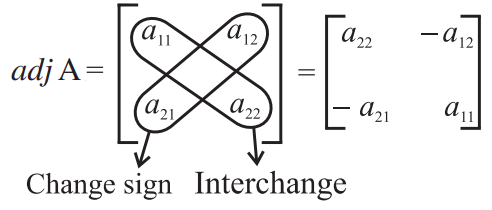Learn how to find the cofactor of elements of a matrix here.

## Adjoint Of a Matrix 3 x 3

Consider a 3 x 3 matrix as:

$A=\begin{bmatrix} a_{11} & a_{12} & a_{13}\\ a_{21} & a_{22} & a_{23}\\ a_{31} & a_{22} & a_{33} \end{bmatrix}$

The adjugate of this matrix is given by:

$\large adj\ A=\begin{bmatrix} A_{11} & A_{12} & A_{13}\\ A_{21} & A_{22} & A_{23}\\ A_{31} & A_{22} & A_{33} \end{bmatrix}^T = \begin{bmatrix} A_{11} & A_{21} & A_{31}\\ A_{12} & A_{22} & A_{32}\\ A_{13} & A_{23} & A_{33} \end{bmatrix}$

Here,

$\begin{bmatrix} A_{11} & A_{12} & A_{13}\\ A_{21} & A_{22} & A_{23}\\ A_{31} & A_{22} & A_{33} \end{bmatrix}$ is the cofactor matrix of A.

The above formula can be expanded as: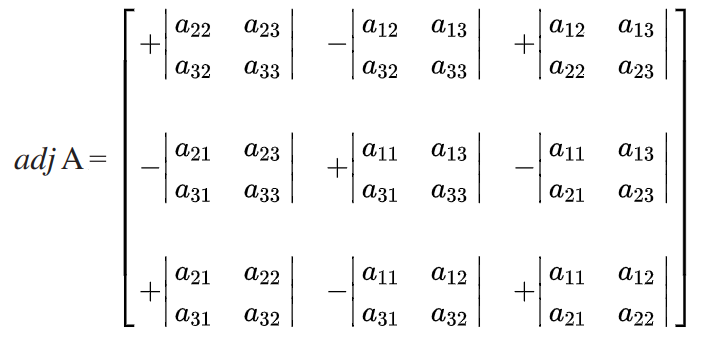Alternatively, we can find the cofactors of the matrix using the formula,

Cofactor of the element aij = Cij = (−1)i+j det(Mij)

Where, det(Mij) is called the minor of aij.

### How To Calculate The Adjoint of a Matrix

We can also write the general formula to find the adjugate of a matrix of order n x n. Let A be the matrix of order n x n, then its adjugate matrix can be written as:

$\large A = \begin{bmatrix} a_{11} & a_{12} & …. & a_{1n}\\ a_{21} & a_{22} & …. & a_{2n}\\ : & : & …. & :\\ : & : & …. & :\\ a_{n1} & a_{n2} & …. & a_{nn} \end{bmatrix}$

Now, the adjoint of this matrix is:

$\large adj\ A = Transpose\ of\ \begin{bmatrix} A_{11} & A_{12} & …. & A_{1n}\\ A_{21} & A_{22} & …. & A_{2n}\\ : & : & …. & :\\ : & : & …. & :\\ A_{n1} & A_{n2} & …. & A_{nn} \end{bmatrix}=\begin{bmatrix} A_{11} & A_{21} & …. & A_{n1}\\ A_{12} & A_{22} & …. & A_{n2}\\ : & : & …. & :\\ : & : & …. & :\\ A_{1n} & A_{2n} & …. & A_{nn} \end{bmatrix}$

Here, A11, A12,…, A21, A22,…., Ann are the cofactors of the elements a11, a12,…, a21, a22,….,ann respectively.

### Adjoint of a Matrix Properties

Some of the important properties of adjugate matrices are listed below.

If A be any given square matrix of order n, we can define the following:

• A(adj A) = (adj A) A = A I, where I is the identity matrix of order n
• For a zero matrix 0, adj(0) = 0
• For an identity matrix I, adj(I) = I
• If A is an invertible matrix and A-1 be its inverse, then:

adj A is invertible with inverse (det A)-1 A

• Suppose A and B are two matrices of order n, then adj(AB) = (adj B)(adj A)
• If A is invertible, then the above formula also holds for negative k.

## Adjoint of a Matrix Examples

Example 1:

Find the adjoint of the matrix $A =\begin{bmatrix} 2 & 3\\ 1 & 4 \end{bmatrix}$.

Solution:

Given,

$A =\begin{bmatrix} 2 & 3\\ 1 & 4 \end{bmatrix}$

Here, a11 = 2, a12 = 3, a21 = 1 and a22 = 4.

So the cofactors are:

A11 = a22 = 4

A12 = -a12 = -3

A21 = -a21 = -1

A22 = a11 = 2

Therefore, $adj\ A =\begin{bmatrix} 4 & -3\\ -1 & 2 \end{bmatrix}$.

Example 2:

Calculate the adjoint of the matrix $adj\ A =\begin{bmatrix} 1 & -1 & 2\\ 2 & 3 & 5\\ 1 & 0 & 3 \end{bmatrix}$.

Solution:

Given,

$adj\ A =\begin{bmatrix} 1 & -1 & 2\\ 2 & 3 & 5\\ 1 & 0 & 3 \end{bmatrix}$

Let Cij be the cofactor of the element aij in matrix A.

Now, the cofactors of the elements of the first row are: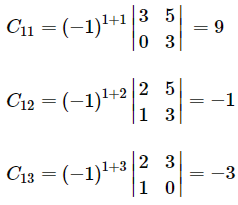Cofactors of second row elements are: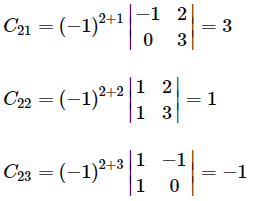Cofactors of third row elements are: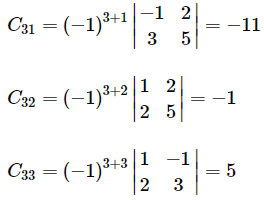The cofactor matrix of A $=\begin{bmatrix} 9 & -1 & -3\\ 3 & 1 & -1\\ -11 & -1 & 5 \end{bmatrix}$

Therefore,

$adj\ A=\begin{bmatrix} 9 & 3 & -11\\ -1 & 1 & -1\\ -3 & -1 & 5 \end{bmatrix}$

The below practice problems based on the adjoint matrix help in better understanding of the concept.

### Adjoint of a Matrix Questions

1. Find the adjoint of the matrix $X=\begin{bmatrix} 1 & 2 & 3\\ 0 & 2 & 4\\ 0 & 0 & 5 \end{bmatrix}$.
2. What is the adjugate matrix of $A=\begin{bmatrix} 1 & 1 & 1\\ 1 & -2 & 3\\ 3 & -1 & 4 \end{bmatrix}$?
3. Calculate the adjoint of $A=\begin{bmatrix} -1 & 5\\ 0 & 3 \end{bmatrix}$.

For more interesting concepts related to matrices, download BYJU’S – The Learning App and learn all the concepts efficiently.